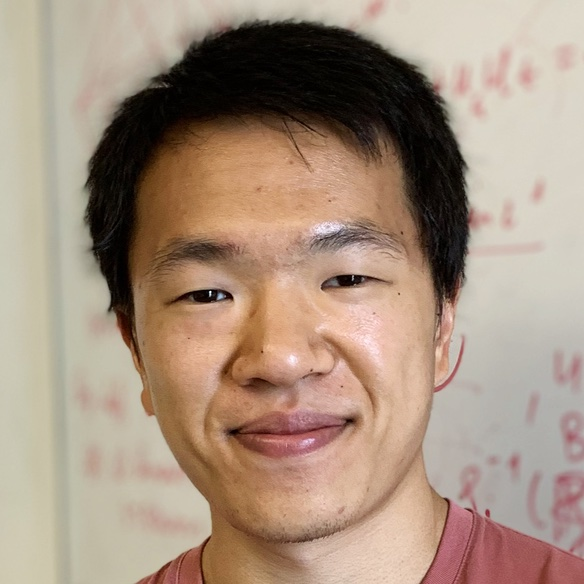# Homepage of Jeremy Wu 🇨🇦

DPhil Student He/Him

S1.07 Mathematical Institute
University of Oxford
United Kingdom

E-mail: jeremy(dot)wu(at)maths(dot)ox(dot)ac(dot)uk

CV

As an applied mathematician, my main interest is the study of Partial Differential Equations (PDE) for modelling physical phenomena. In particular, the focus of my thesis is on the gradient flow structure of the spatially homogeneous Landau-Fokker-Planck equation $$\partial_t f(t,v) = \nabla \cdot \left(f(v)\int_{\mathbb{R}^3}f(v_*) |v-v_*|^{2+\gamma} \left[I - \frac{v \otimes v}{|v|^2} \right](\nabla \log f(v) - \nabla \log f(v_*))dv_* \right), \quad \gamma \in [-4,0].$$